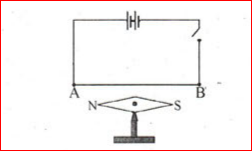# Kerala Class 9 Physics Important Questions

Kerala Class 9 Physics Important Questions is very useful as it helps students to prepare for the exams ahead. Other study materials the class 9 students use include the SCERT books for Class 9, which also lays the foundation for these important questions.

See, here the various topics that are covered for Class 9 Physics as per the Kerala Syllabus 9th standard. From the nature of minerals to Motion, Wave motion and Gravitation or Work, Energy, Power, the important questions cover all the important points.

Also, you can get the list of important questions below:

1. Hydrometer is an instrument used to measure the relative density of a liquid.

1. Write the working principle of hydrometer.
2. Why do the values of markings on the hydrometer increase towards the bottom?

2. Artificial satellites are classified into Equatorial satellites and polar satellites.

1. Write any one use of each type of satellite
2. What is the condition for a Equatorial satellite to behave as a geostationary satellite?

3. A conductor is arranged parallel to a pivoted magnetic needle as in the figure:1. When current flows through AB, the magnetic needle deflects. What is the reason?
2. Write any one method to reverse the deflection of the magnetic needle.
3. Name and state the law which helped you to find the direction of the deflection of the north pole of the magnetic needle?

4. Explain why big boulders are carried by fast moving rivers, over hundreds of kilometers.

5. Explain why ice-bergs floating in seas are dangerous for ships.

6. A wooden block floats in water with 2/3rd of the volume submerged.

1. Calculate the density of volume
2. When the same block is placed in oil, three-quarters of its volume is immersed in oil. Calculate the density of oil.

7. Write symbols and state functions of each of the following components in an electric circuit.

1. Rheostat (b) Volt meter

8. Define the S.I Unit of pressure.

9. Draw a displacement- time graph for a boy going to school with a uniform velocity.

10. The thimble of a screw gauge has 50 divisions. The spindle advances 2mm when the screw is turned through four revolutions.

1. What is the pitch of the screw gauge?
2. What is the least count of the screw gauge?

11. Two pendulums P and Q have equal lengths but their bobs weigh 10gF and 20gF respectively.

1. Compare their time periods

12. If I travel from Mumbai to Pune (150km) in 2 and ½ hours via the express highway, and return to mumbai via old highway (180km) in 3 and ½ hours. Calculate the average velocity during the entire journey.

13. Write any two properties of magnetic field lines

14. State Ohm’s Law

15. Differentiate primary cells and secondary cells, in terms of:

1. Internal Resistance (b) Energy Changes

16. What is an electromagnet? Draw a labelled diagram to make an electromagnet from a soft iron bar. Mark the polarity at its ends.

17. (a) State Archimedes Principle

(b) It is easier to lift a heavy stone under water than in air. Explain

18. Differentiate between scalar and vector quantities. Give one example of each.

19. State 2 technological measure to minimize the impact of global warming.

20. Draw a ray diagram to illustrate how a ray of light incident obliquely on one face of a rectangular glass slab of uniform thickness emerges.

If students wish to get more details about Kerala Board Class 9 and study materials, they can also reach out to BYJU’s.